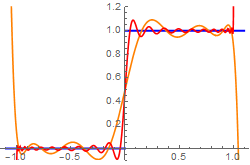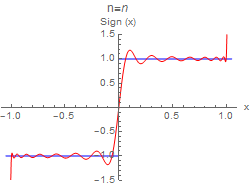# Preface

This section presents the basic properties of solutions to the Laplace and Helmholtz equations.

Introduction to Linear Algebra with Mathematica

# Legendre Polynomials

Adrien-Marie Legendre (1752--1833) was a French mathematician. Legendre made numerous contributions to mathematics. Well-known and important concepts such as the Legendre polynomials and Legendre transformation are named after him.

Consider Legendre's equation

$\left( 1-x^2\right) y'' -2x\,y' + n(n+1)\, y =0 ,$
which can be rewritten in self-adjoint form
$\frac{\text d}{{\text d}x} \left[ \left( 1- x^2 \right) \frac{{\text d} y}{{\text d}x} \right] + n(n+1)\, y =0 ,$
where n is a real constant, but it is usually an integer. The above equation is named after a French mathematician Adrien-Marie Legendre (1752--1833) who introduced the Legendre polynomials in 1782. Legendre's equation comes up in many physical situations involving spherical symmetry. When n is an integer, the Legandre differential equation has a polynomial solution (with the normalization $$P_n (1) =1$$ ) that is usually denoted by $$P_n (x)$$ and is called Legendre's polynomial of order n. The Legendre equation has non-polynomial solutions represented by infinite series. These are the Legendre functions of the second kind, denoted by $$Q_n (x) .$$ Each Legendre polynomial may be expressed using Rodrigues' formula (named after Benjamin Olinde Rodrigues (1795--1851), a French banker, mathematician, and social reformer):
$P_n (x) = \frac{1}{2^n n!} \,\frac{{\text d}^n}{{\text d}x^n} \left( x^2 -1 \right)^n , \qquad n=1,2,\ldots .$
They can also be defined via Bonnet’s recursion formula:
$\left( n+1 \right) P_{n+1} (x) = \left( 2n+1 \right) x\, P_n (x) - n\,P_{n-1} (x), \qquad n=2,3, \ldots ; \quad P_0 (x) =1, \quad P_1 (x) =x;$
which follow from the generating function for Legendre polynomials:
$\frac{1}{\sqrt{1- 2xt + t^2}} = \sum_{n\ge 0} P_n (x)\, t^n \qquad\Longrightarrow \qquad P_n (x) = \frac{1}{2^n} \,\sum_{k=0}^n \binom{n}{k}^2 \left( x-1 \right)^{n-k} \left( x+1 \right)^k .$
Its exponential generating function is
$e^{xz}\,J_0 \left( z \sqrt{1-x^2} \right) = \sum_{n\ge 0} P_n (x)\, \frac{z^n}{n!} .$
The Rodrigues formula can be derived from the Lagrange inversion theorem (LIT) using
$w = f(z) = 2 \, \frac{z- z_0}{z^2 -1} ,$
where $$z_0 = z(0) ,$$ with $$w_0 = f(z_0 ) .$$ Then
$\frac{1}{1 - (w- w_0 ) \, \frac{{\text d}}{{\text d} x} \left( \frac{z-z_0}{f(z) - w_0} \right)_{z=z(w)}} = \sum_{n\ge 0} c_n \left( w- w_0 \right)^n ,$
where
$c_n = \frac{1}{n!} \, \frac{{\text d}^n}{{\text d} z^n} \left. \left( \frac{z- z_0}{f(z) - w_0} \right) \right\vert_{z= z_0} = \frac{1}{2^n \, n!} \, \frac{{\text d}^n}{{\text d} z^n} \left( z^2 -1 \right)^n .$
Legendre polynomials are eigenfunctions of a singular Sturm–Liouville problem:
$\frac{\text d}{{\text d}x} \left[ \left( 1- x^2 \right) \frac{{\text d} y}{{\text d}x} \right] = - \lambda\, y , \qquad y(\pm 1) < \infty ,$
where the eigenvalue λ corresponds to n(n + 1).

Legendre polynomials are symmetric or antisymmetric, that is,

$P_n (-x) = (-1)^n P_n (x) , \qquad n=0,1,2,\ldots .$
We remind the numerical values:
$P_n (1) = 1 , \qquad P_n (-1) = (-1)^n , \qquad P_{2n} (0) = (-1)^n \,\frac{(2n)!}{2^{2n} \, (n! )^2} ,\qquad P'_{n} (1) = \frac{n(n+1)}{2} , \qquad n=0,1,2,\ldots .$
The Legendre polynomials are built into Mathematica. Mathematica's notation is LegendreP[n,x] for $$P_n (x).$$ We now use Mathematica to obtain the formulas for the first 11 of these polynomials. We put them in a table.
TableForm[Table[{i, i*(i + 1), LegendreP[i, x]}, {i, 0, 10}], TableHeadings -> {None, {"n", "$Lambda]_k", "P_n (x)"}}] To get some idea of what these polynomials look like, we construct graphs of the first 7. We first define a function legraph[n] that produces a graph of the kth polynomial, and then we use a Do loop to construct the first 7 graphs. legraph[n_] := Plot[LegendreP[n, x], {x, -1, 1}, AxesLabel -> {"x", "P_n (x)"}, PlotRange -> {{-1.1, 1.1}, {-1.1, 1.1}}, PlotLabel -> Row[{"n=", PaddedForm[n, 3]}]] GraphicsGrid[Table[{legraph[i], legraph[i + 1]}, {i, 0, 6, 2}]] First we set up a series to work with: y = Sum[c[i] x^i, {i, 0, 6}] + O[x]^7 Out= c + c x + c x^2 + c x^3 + c x^4 + c x^5 + c x^6 + O[x]^7 To see that our input is correct we type OutputForm[y]//Normal We are going to insert this series into the differential equation de = (1 -x^2) D[y, {x,2}] - 2 x D[y,x] + 20 y == 0 Out= (20 c + 2 c) + (18 c + 6 c) x + (14 c + 12 c) x^2 + (8 c + 20 c) x^3 + 30 c x^4 + O[x]^5 == 0 Then we get the coefficients coeffeqns = LogicalExpand[de] Out= 20 c + 2 c == 0 && 18 c + 6 c == 0 && 14 c + 12 c == 0 && 8 c + 20 c == 0 && 30 c == 0 and express through first two c and c: solvedcoeffs = Solve[ coeffeqns, Table[ c[i], {i,2,12}]] Solve::svars: Equations may not give solutions for all "solve" variables. >> Out= {{c -> -10 c, c -> -3 c, c -> (35 c)/3, c -> (6 c)/5, c -> 0}} Put these back into the series expansion. y = y /. solvedcoeffs Out= { c + c x -10 c x^2 - 3 c x^3 + (35/3) c x^4 + (6/5) c x^5 + O[x]^7 } Extract out the two linearly independent solutions Coefficient[ y, c] Out= {1 - 10 x^2 + (35 x^4)/3} Coefficient[ y, c] Out= {x - 3 x^3 + (6 x^5)/5} To check our answer, we use Mathematica again because it knows Legendre: LegendreP[4,x] Out= 1/8 (3 - 30 x^2 + 35 x^4) This is different from the polynomial obtained earlier, but only by a constant factor. The constant factor is used to set the normailization for the Legendre polynomials. # Legendre Expansions Since Legendre polynomial are orthogonal \[ \int_{-1}^1 P_n (x) \, P_m (x) \,{\text d} x = 0 \qquad\mbox{for} \quad n\ne m,$
any suitable function $$f (x)$$ on the interval [-1,1] can be expanded into generalized Fourier--Legendre series:
$f(x) = \sum_{n\ge 0} c_n P_n (x) ,$
where the coefficients are
$c_n = \left( n + \frac{1}{2} \right) \int_{-1}^1 P_n (x) \, f(x) \,{\text d} x , \qquad n=0,1, \ldots ,$
because
$\int_{-1}^1 P_n^2 (x) \,{\text d} x = \frac{2}{2n+1} .$

Example 1: Find the Fourier-Legendre series expansion of the Heaviside step function H(t), defined on the finite interval [-1,1].

Its series expansion is written in the form

$H(t) = \sum_{n\ge 0} c_n \,P_n (t) , \qquad t \in [-1,1] .$
Substituting the explicit expressions for the Legendre polynomials, we get
\begin{align*} c_n &= \frac{2n+1}{2} \, \int_{-1}^1 H(t)\, P_n (t)\,{\text d}t = \frac{2n+1}{2} \, \int_{0}^1 \, P_n (t)\,{\text d}t . \end{align*}
Integrating by parts, we find the explicit expressions for the coefficients:
\begin{align*} c_n &= \frac{2n+1}{2} \,\frac{1}{2^n \,n!} \int_{0}^1 \frac{{\text d}^n}{{\text d} t^n} \left( t^2 -1 \right)^n {\text d}t = \frac{2n+1}{2^{n+1} n!} \, \left[ \frac{{\text d}^{n-1}}{{\text d} t^{n-1}} \left( t^2 -1 \right)^n \right]_{t=0}^{t=1} . \\ &= \left\{ \begin{array}{ll} 0 , & \ \mbox{if $n$ is even} \\ (-1)^k \,\frac{(4k+3) \,(2k)!}{2^{2k+2}\,k! \,(k+1)!} , & \ \mbox{if $n = 2k+1$ is odd. } \end{array} \right. \end{align*}
Thus, the Fourier--Legendre series expansion of the Heaviside function is given by
$H(t) = \frac{1}{2} + \sum_{k\ge 0} \, \frac{(-1)^k \,(4k+3) \,(2k)!}{2^{2k+2}\,k! \,(k+1)!} \, P_{2k+1} (x) .$
Then we plot finite approximations with 5 and 20 terms:
legendre5 = 1/2 + Sum[(-1)^k *(4*k + 3)* Factorial[2*k]/2^(2*k + 2)/Factorial[k + 1]/Factorial[k] * LegendreP[2*k + 1, t], {k, 0, 5}];
legendre20 = 1/2 + Sum[(-1)^k *(4*k + 3)* Factorial[2*k]/2^(2*k + 2)/Factorial[k + 1]/Factorial[k] * LegendreP[2*k + 1, t], {k, 0, 20}];
Plot[{HeavisideTheta[t], legendre5, legendre20}, {t, -1.1, 1.1}, PlotStyle -> {{Blue, Thick}, Orange, Red}, PlotRange -> {-0.1, 1.2}]Example 2: Suppose we want to expand the signum function into the Fourier--Legendre series. Using an integration formula

$\int_{-1}^x P_n (t) \,{\text d}t = \frac{P_{n+1} (x) - P_{n-1} (x)}{2n+1} ,$
we find the explicit expressions for the coefficients:
$c_n = \frac{2n+1}{2}\,\int_{-1}^i \mbox{sign}(x)\, P_n (x) \,{\text d}x = P_{n-1} (0) - P_{n+1} (0) , \qquad n=1,2,\ldots ,$
where $$P_{2k+1} (0) =0$$ and formulas for even indices was given previously. We now use Mathematica to calculate the coefficients up to arbitrary n, starting with n = 1.
Module[{i}, coef = {}; Do[coef = Append[coef, (LegendreP[i - 1, 0] - LegendreP[i + 1, 0])], {i, 1, 30}]]
The nth coefficient is then given by coef[[n]]. We sample a few values:
coef[]
Out= 180557/524288
coef[]
Out= 0
All the even coefficients are zero.

Now we define the mth partial sum, and then a graph of the mth partial sum, along with the original function. Because the coefficient of $$P_{0} (x)=1$$ is zero, we may start the sum over n with the n = 1 term. The given signum function is shown in blue and the partial sums in red.

legsum[m_, x_] := Module[{i}, Sum[N[coef[[i]]]*LegendreP[i, x], {i, 1, m}]]
legraph[m_] := Plot[{Sign[x], legsum[m, x]}, {x, -1.05, 1.05}, AxesLabel -> {"x", "Sign (x)"}, PlotRange -> {-1.5, 1.5}, PlotStyle -> {{RGBColor[0, 0, 1], Thickness[0.005]}, {RGBColor[1, 0, 0], Thickness [0.005]}}, PlotLabel -> Row[{"n=", PaddedForm[n, 3]}]]
legraphYou may want to construct a sequence of partial sums which may be animated to see the convergence:
DynamicModule[{mangraph, i}, Do[mangraph[i] = legraph[i], {i, 1, 55, 2}]; Manipulate[mangraph[i], {i, 1, 51, 2}]]
You can use the slider to move through the graphs, and also to display a movie of the graphs. When you do this, you will see the familiar Gibbs overshoot/undershoot at the discontinuity. It is also observed a struggle to converge at the endpoints.
Module[{a}, coef = {}; Do[coef = Append[coef, (LegendreP[x - 1, 0] - LegendreP[x + 1, 0])], {x, 1, 100}]] legsum[m_, x_] := Module[{a}, -Sum[N[coef[[a]]]*LegendreP[a, x], {a, 1, m}]] leg[x_] = Piecewise[{{1, -1 <= x < 0}, {-1, 0 < x <= 1}}]; legraph[m_] := Plot[{leg[x], legsum[m, x]}, {x, -1, 1}, PlotRange -> {-1.5, 1.5}, PlotStyle -> {{RGBColor[0, 0, 1], Thickness[0.005]}, {RGBColor[1, 0, 0], Thickness[0.005]}}] legraph legraph

Example 3: The Dirac delta function admits the following Fourier--Legendre expansion:

$\delta (x-a) = \sum_{k\ge 0} \left( k + \frac{1}{2} \right) P_k (x)\, P_k (a) .$
or
$\delta (x-a) = \frac{1}{2} \sum_{k\ge 0} \left( 2k+1 \right) P_k (x)\, P_k (a) .$
So the partial sums
$\delta_N (x-a) = \frac{1}{2} \sum_{k= 0}^{N} \left( 2k+1 \right) P_k (x)\, P_k (a) \qquad \mbox{for} \quad |x| < 1,$
have the property:
$\lim_{N\to \infty} \int_{-1}^1 {\text d}x \, \delta_N (x-a) \,f(x) = f(a) .$
■